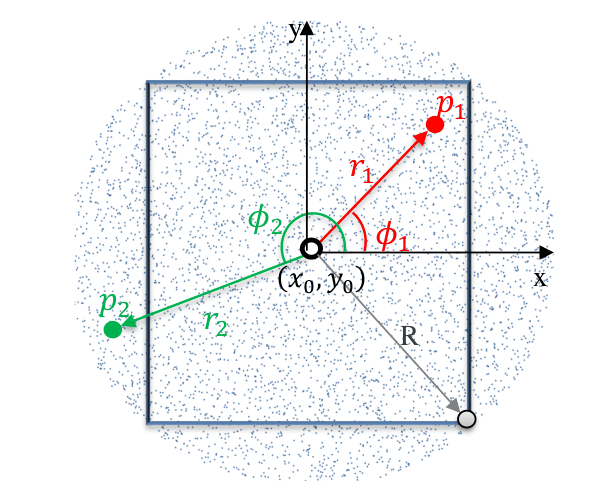# Distribution of random rays

## 1. Distribution of point source of ray

To have more precision, the point source is random in element. A element is triangle of origin point $P$ and directional vector $\mathbf{e_1}$ and $\mathbf{e_2}$.

We choose the point in circumscribed circle of center $C = P + \frac{1}{3} \mathbf{e_1} + \frac{1}{3} \mathbf{e_2} = \begin{pmatrix} x_C \\ y_C \end{pmatrix}$ and ray $R = ||\vec{CP}|| = \frac{1}{3} || \mathbf{e_1} + \mathbf{e_2}||$.Figure 1. Random generation of source point, see [Ozturk]

The coordinate of a source point $S = (x_i,y_i)$ can be computed as follows as described in [Ozturk]:

$\begin{eqnarray} \phi_i &=& 2\pi \xi_1 \\ r_i &=& R \sqrt{\xi_2} \\ x_i &=& x_C + r_1 cos \phi_i \\ y_i &=& y_C + r_i sin \phi_i \end{eqnarray}$

With $\xi_1$ and $\xi_2$ is choose with uniformely distribution in $[0,1$].

The source point can be not in the element, thus we remove the source point and we choose an other source point until to have a source point in the finite element.

To know if $S$ is in element, we have the condition :

$Q \in \mathcal{T} \Longleftrightarrow \left\{ \begin{eqnarray} 0 \leq u \leq 1 \\ 0 \leq v \leq 1 \\ u + v \leq 1 \end{eqnarray} \right.$

With :

$\begin{eqnarray} u &=& \frac{(\mathbf{e_2} \cdot \mathbf{e_2}) (\vec{PQ} \cdot \mathbf{e_1}) - (\mathbf{e_1} \cdot \mathbf{e_2}) (\vec{PQ} \cdot \mathbf{e_2}) }{(\mathbf{e_1} \cdot \mathbf{e_1})(\mathbf{e_2} \cdot \mathbf{e_2})-(\mathbf{e_1} \cdot \mathbf{e_2})^2} \\ v &=& \frac{(\mathbf{e_1} \cdot \mathbf{e_1}) (\vec{PQ} \cdot \mathbf{e_2}) - (\mathbf{e_1} \cdot \mathbf{e_2}) (\vec{PQ} \cdot \mathbf{e_1}) }{(\mathbf{e_1} \cdot \mathbf{e_1})(\mathbf{e_2} \cdot \mathbf{e_2})-(\mathbf{e_1} \cdot \mathbf{e_2})^2} \end{eqnarray}$

The algorithme to create source point is :

```\begin{algorithm}
\caption{Source Point}
\begin{algorithmic}
\FUNCTION{SourcePoint}{$\mathcal{T}$}
\STATE $\mathcal{T}$ triangle of origin point $P$ and directional vectors $\mathbf{e_1}$ and $\mathbf{e_2}$

\STATE $\xi_1$ and $\xi_2$ random number in $[0,1]$
\STATE $\phi \leftarrow 2\pi \xi_1$
\STATE $r \leftarrow R \sqrt{\xi_2}$
\STATE $Q \leftarrow \begin{pmatrix} x_C + r cos \phi \\ y_C + r sin \phi \end{pmatrix}$

\WHILE{$Q$ isn't in $\mathcal{T}$}
\STATE $\xi_1$ and $\xi_2$ random number in $[0,1]$
\STATE $\phi \leftarrow 2\pi \xi_1$
\STATE $r \leftarrow R \sqrt{\xi_2}$
\STATE $Q \leftarrow \begin{pmatrix} x_C + r cos \phi \\ y_C + r sin \phi \end{pmatrix}$
\ENDWHILE
\RETURN $Q$
\ENDFUNCTION
\end{algorithmic}
\end{algorithm}
```

## 2. Distribution of direction

We see in foreign section, the diffusion (or absorptive or reflexion or transmission) of rays depends on incident angle (perhaps azimuthal angle) and on wavelenght.

The goal of section is to find a good distribution of wavelenght, incident angle and azimuthal angle. We calculate the inverse of repartition fonction.

### 2.1. Distribution of wavelenght

$R_{\lambda} = \frac{1}{\epsilon \sigma T^4} \int_{\lambda'=0}^{\lambda}{\epsilon_{\lambda'} E_{b\lambda'} d\lambda'}$

We take the reciprocal : $\lambda = \lambda(R_{\lambda})$

To have a random $\lambda$, we draw a random number $R_{\lambda}$ and calculate $\lambda$ with it.

If the matters of emitter surface is black, we can simplify :

$R_{\lambda} = \frac{1}{\sigma T^4} \int_{\lambda'=0}^{\lambda}{E_{b\lambda'}d\lambda'} = f(\lambda T)$

If the matters of emitter surface is gray, we can simplify :

$R_{\lambda} = \frac{1}{\sigma T^4} \int_{\lambda'=0}^{\lambda}{E_{b\lambda'}d\lambda'} = f(\lambda T)$

### 2.2. Distribution of incident angle of emission

The spectral emissive power is :

$E_{\lambda} = \int_{2\pi}{ \epsilon'_{\lambda} I_{b\lambda} cos \theta d\Omega} = \frac{E_{b\lambda}}{\pi} \int_{\psi=0}^{2\pi}{ \int_{\theta'=0}^{\pi/2}{ \epsilon'_{\lambda} cos \theta' sin \theta' d\theta'} d\psi'}$
$R_{\psi} = \frac{1}{\pi} \int_{\psi'=0}^{\psi}{ \int_{\theta'=0}^{\theta}{\frac{\epsilon'_{\lambda}}{\epsilon_{\lambda}} cos \theta' sin \theta' d\theta} d\psi }$

We take the reciprocal : $\psi = \psi(R_{\psi})$

To have a random $\psi$, we draw a random number $R_{\psi}$ and calculate $\psi$ with it.

Most surfaces tand to be isotropic so that the directional emittance does not depend on azimithal angle $\psi$, we have simplification :

$R_{\psi} = \frac{\psi}{2\pi} \text{ or } \psi = 2\pi R_{\psi}$

### 2.3. Distribution of azimuthal angle of emission

$R_{\theta} = \frac{\int_{\theta'=0}^{\theta}{\epsilon'_{\lambda} cos \theta' sin \theta' d\theta'}}{\int_{\theta'=0}^{\pi/2}{\epsilon'_{\lambda} cos \theta' sin \theta' d\theta'}}$

We take the reciprocal : $\theta = \theta(R_{\theta})$

To have a random $\theta$, we draw a random number $R_{\theta}$ and calculate $\theta$ with it.

For a diffuse emitter, we have simplification :

$R_{\theta} = sin^2 \theta \text{ or } \theta = arcsin \sqrt{R_{\theta}}$

Or an other form :

$R_{\theta} = \frac{1-cos(2\theta)}{2} \text{ or } \theta = \frac{1}{2} arccos(1-2R_{\theta})$

### 2.4. Distribution of incidence angle of reflection

$\begin{eqnarray} {\rho'}_{\lambda}^{\nabla}(\lambda, \theta_i, \psi_i) &=& \int_{2\pi}{\rho_{\lambda}''(\lambda, \theta_i, \psi_i, \theta_r, \psi_r) cos \theta_r d\Omega_r} \\ &=& \int_{\psi_r=0}^{2\pi}{ \int_{\theta_r=0}^{\pi/2}{ {\rho''}_{\lambda}(\lambda, \theta_i, \psi_i, \theta_r, \psi_r) d\theta_r} d\psi_r} \end{eqnarray}$
$R_{\psi'} = \frac{1}{{\rho'}^{\nabla}_{\lambda}} \int_{}^{}{ \int_{}^{}{} }$

If the surface is a diffuse reflector, we have ${\rho''}_{\lambda}(\lambda, \theta_i, \psi_i, \theta_r, \psi_r) = {\rho''}_{\lambda} = \frac{{\rho'}^{\nabla}_{\lambda}(\lambda)}{\pi}$, we simplify :

$R_{\psi} = \frac{\psi_r}{2\pi} \text{, or } \psi_r = 2\pi R_{\psi}$

## References

• [Incropera] Fundamentals of Heat and Mass Transfer, Theodore L. Bergman, Adrienne S. Lavine, Frank P. Incropera, David P. DeWitt, Wiley.

• [Kumaran1996] Kumaran, M. Kumar, Heat, Air and Moisture Transfer in Insulated Envelope Parts, Final Report, Volume 3.

• [Ozturk] Abdurrahman Ozturk, Implementation of View Factor Model and Radiative Heat Transfer Model in MOOSE. University of South Carolina, Download PDF

• [Modest] M.F. Modest, Radiative Heat Transfet. Elsevier Science, 2013, ISBN 9780123869906, Chapter 8

• [Visionary] Visionaray: A Cross-Platform Ray Tracing Template Library, Zellmann, Stefan and Wickeroth, Daniel and Lang, Ulrich, Proceedings of the 10th Workshop on Software Engineering and Architectures for Realtime Interactive Systems (IEEE SEARIS 2017), 2017

• [Visionary::github] Visionaray, Zellman, github.com/szellmann/visionaray